# 聚类算法之Affinity Propagation(AP)

## Affinity Propagation算法简介

AP(Affinity Propagation)通常被翻译为近邻传播算法或者亲和力传播算法。AP算法的基本思想是将全部数据点都当作潜在的聚类中心(称之为exemplar)，然后数据点两两之间连线构成一个网络(相似度矩阵)，再通过网络中各条边的消息(responsibility和availability)传递计算出各样本的聚类中心。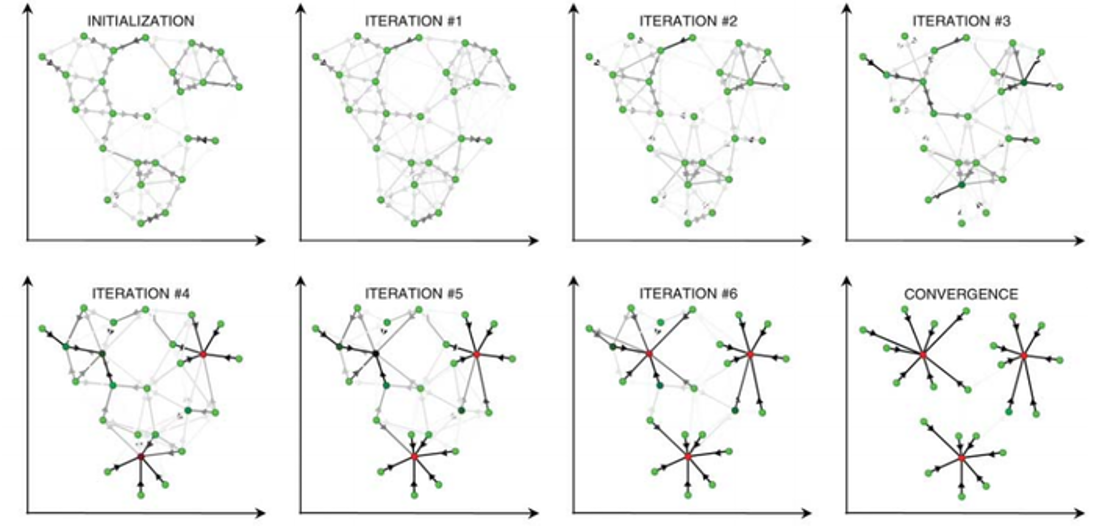AP算法中的特殊名词：

• Exemplar：指的是聚类中心，K-Means中的质心。
• Similarity（相似度）：点j作为点i的聚类中心的能力，记为S(i,j)。一般使用负的欧式距离，所以S(i,j)越大，表示两个点距离越近，相似度也就越高。使用负的欧式距离，相似度是对称的，如果采用其他算法，相似度可能就不是对称的。
• Preference：指点i作为聚类中心的参考度(不能为0)，取值为S对角线的值(图1红色标注部分)，此值越大，最为聚类中心的可能性就越大。但是对角线的值为0，所以需要重新设置对角线的值，既可以根据实际情况设置不同的值，也可以设置成同一值。一般设置为S相似度值的中值。
• Responsibility（吸引度）：指点k适合作为数据点i的聚类中心的程度，记为r(i,k)。如图2红色箭头所示，表示点i给点k发送信息，是一个点i选点k的过程。
• Availability(归属度)：指点i选择点k作为其聚类中心的适合程度，记为a(i,k)。如图3红色箭头所示，表示点k给点i发送信息，是一个点k选点i的过程。
• Damping factor(阻尼系数)：主要是起收敛作用的。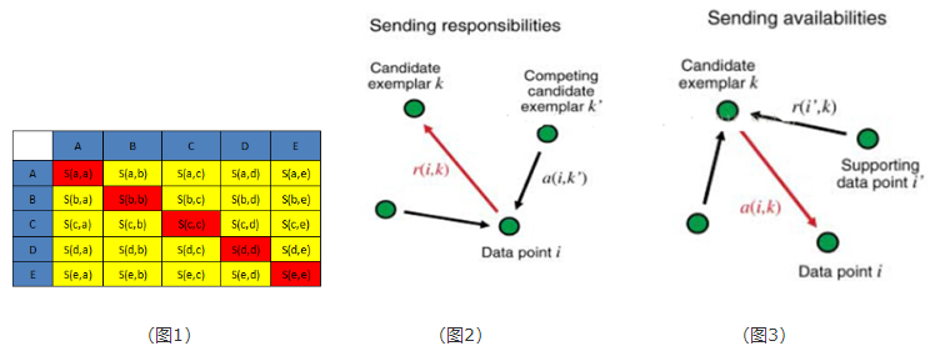AP算法流程：

• 步骤1：算法初始，将吸引度矩阵R和归属度矩阵初始化为0矩阵；
• 步骤2：更新吸引度矩阵

$$r_{t+1}(i, k)=\{\begin{array}{l}{S(i, k)-\max _{j \neq k}\left\{a_{t}(i, j)+r_{t}(i, j)\right\}, i \neq k} \\ {S(i, k)-\max _{j \neq k}\{S(i, j)\}, i=k} \end{array}$$

• 步骤3：更新归属度矩阵步骤4：根据衰减系数 对两个公式进行衰减

$$a_{t+1}(i, k)=\{\begin{array}{l}{\min \left\{0, r_{t+1}(k, k)+\sum_{j \neq i, k} \max \left\{r_{t+1}(j, k), 0\right\}\right\}, i \neq k} \\ {\sum_{j \neq k} \max \left\{r_{t+1}(j, k), 0\right\}, i=k} \end{array}$$

• 步骤4：根据衰减系数$\lambda$对两个公式进行衰减

\begin{aligned} &r_{t+1}(i, k)=\lambda * r_{t}(i, k)+(1-\lambda) * r_{t+1}(i, k)\\ &a_{t+1}(i, k)=\lambda * a_{t}(i, k)+(1-\lambda) * a_{t+1}(i, k) \end{aligned}

• 重复步骤2，3,4直至矩阵稳定或者达到最大迭代次数，算法结束。
• 最终取a+r最大的k作为聚类中心。

## Python下AP算法使用

Python的机器学习库sklearn中已经实现了AP算法，可以直接调用。

class sklearn.cluster.AffinityPropagation(damping=0.5, max_iter=200, convergence_iter=15, copy=True, preference=None, affinity='euclidean', verbose=False)

• damping : 衰减系数，默认为5
• convergence_iter : 迭代次后聚类中心没有变化，算法结束，默认为
• max_iter : 最大迭代次数，默认
• copy : 是否在元数据上进行计算，默认True，在复制后的数据上进行计算。
• preference : S的对角线上的值
• affinity :S矩阵（相似度），默认为euclidean（欧氏距离）矩阵，即对传入的X计算距离矩阵，也可以设置为precomputed，那么X就作为相似度矩阵。

• cluster_centers_indices_ : 聚类中心的位置
• cluster_centers_ : 聚类中心
• labels_ : 类标签
• affinity_matrix_ : 最后输出的A矩阵
• n_iter_ ：迭代次数

AP（Affinity Propagation）算法演示：

from sklearn.cluster import AffinityPropagation
from sklearn import metrics
from sklearn.datasets.samples_generator import make_blobs
import numpy as np

# 生成测试数据
centers = [[1, 1], [-1, -1], [1, -1]]
X, labels_true = make_blobs(n_samples=300, centers=centers, cluster_std=0.5, random_state=0)

# AP模型拟合
af = AffinityPropagation(preference=-50).fit(X)
cluster_centers_indices = af.cluster_centers_indices_
labels = af.labels_
new_X = np.column_stack((X, labels))

n_clusters_ = len(cluster_centers_indices)

print('Estimated number of clusters: %d' % n_clusters_)
print("Homogeneity: %0.3f" % metrics.homogeneity_score(labels_true, labels))
print("Completeness: %0.3f" % metrics.completeness_score(labels_true, labels))
print("V-measure: %0.3f" % metrics.v_measure_score(labels_true, labels))
print("Silhouette Coefficient: %0.3f"
% metrics.silhouette_score(X, labels, metric='sqeuclidean'))
print('Top 10 sapmles:', new_X[:10])

# 图形展示
import matplotlib.pyplot as plt
from itertools import cycle

plt.close('all')
plt.figure(1)
plt.clf()

colors = cycle('bgrcmykbgrcmykbgrcmykbgrcmyk')
for k, col in zip(range(n_clusters_), colors):
class_members = labels == k
cluster_center = X[cluster_centers_indices[k]]
plt.plot(X[class_members, 0], X[class_members, 1], col + '.')
plt.plot(cluster_center, cluster_center, 'o', markerfacecolor=col,
markeredgecolor='k', markersize=14)
for x in X[class_members]:
plt.plot([cluster_center, x], [cluster_center, x], col)

plt.title('Estimated number of clusters: %d' % n_clusters_)
plt.show()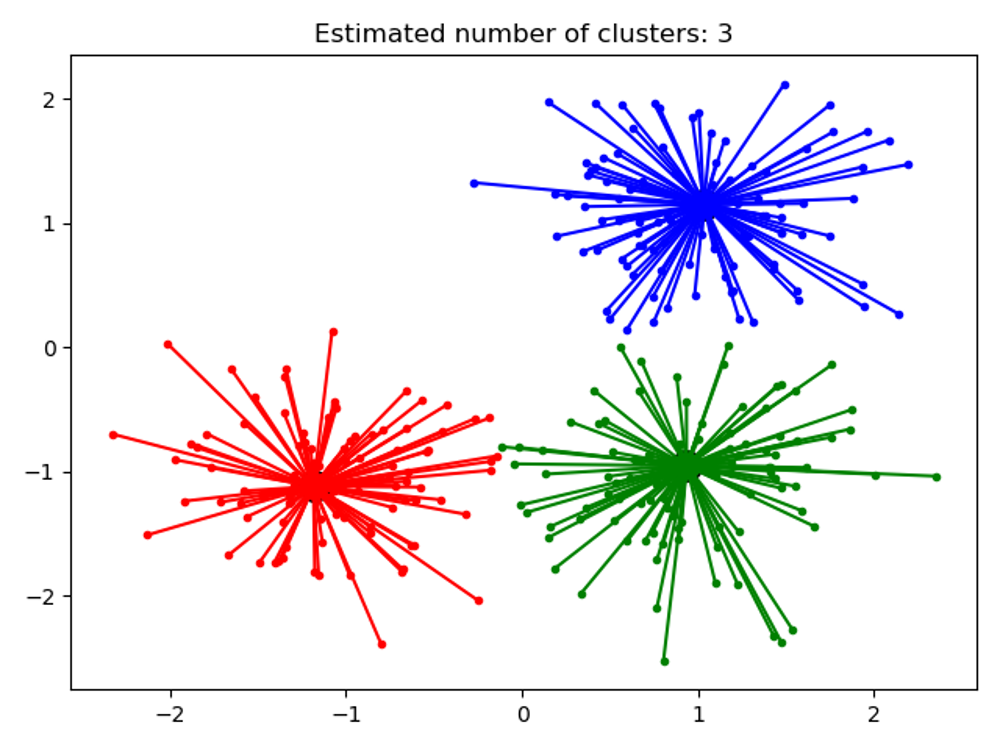## AP与K-Means对比

AP聚类算法与经典的K-Means聚类算法相比，具有很多独特之处：

• 无需指定聚类“数量”参数。AP聚类不需要指定K（经典的K-Means）或者是其他描述聚类个数（SOM中的网络结构和规模）的参数，这使得先验经验成为应用的非必需条件，人群应用范围增加。
• 明确的质心（聚类中心点）。样本中的所有数据点都可能成为AP算法中的质心，叫做Examplar，而不是由多个数据点求平均而得到的聚类中心（如K-Means）。
• 对距离矩阵的对称性没要求。AP通过输入相似度矩阵来启动算法，因此允许数据呈非对称，数据适用范围非常大。
• 初始值不敏感。多次执行AP聚类算法，得到的结果是完全一样的，即不需要进行随机选取初值步骤（还是对比K-Means的随机初始值）。
• 算法复杂度较高，为O(N*N*logN)，而K-Means只是O(N*K)的复杂度。因此当N比较大时(N>3000)，AP聚类算法往往需要算很久。
• 若以误差平方和来衡量算法间的优劣，AP聚类比其他方法的误差平方和都要低。（无论k-center clustering重复多少次，都达不到AP那么低的误差平方和）

AP算法相对K-Means鲁棒性强且准确度较高，但没有任何一个算法是完美的，AP聚类算法的主要缺点：

• AP聚类应用中需要手动指定Preference和Damping factor，这其实是原有的聚类“数量”控制的变体。
• 算法较慢。由于AP算法复杂度较高，运行时间相对K-Means长，这会使得尤其在海量数据下运行时耗费的时间很多。

AP和K-Means运行时间对比

import numpy as np
import matplotlib.pyplot as plt
import time
from sklearn.cluster import KMeans, AffinityPropagation
from sklearn.datasets.samples_generator import make_blobs

# 生成测试数据
np.random.seed(0)
centers = [[1, 1], [-1, -1], [1, -1]]
kmeans_time = []
ap_time = []
for n in [100, 500, 1000]:
X, labels_true = make_blobs(n_samples=n, centers=centers, cluster_std=0.7)

# 计算K-Means算法时间
k_means = KMeans(init='k-means++', n_clusters=3, n_init=10)
t0 = time.time()
k_means.fit(X)
kmeans_time.append([n, (time.time() - t0)])

# 计算AP算法时间
ap = AffinityPropagation()
t0 = time.time()
ap.fit(X)
ap_time.append([n, (time.time() - t0)])

print('K-Means time', kmeans_time[:10])
print('AP time', ap_time[:10])

# 图形展示
km_mat = np.array(kmeans_time)
ap_mat = np.array(ap_time)
plt.figure()
plt.bar(np.arange(3), km_mat[:, 1], width=0.3, color='b', label='K-Means', log='True')
plt.bar(np.arange(3) + 0.3, ap_mat[:, 1], width=0.3, color='g', label='AffinityPropagation', log='True')
plt.xlabel('Sample Number')
plt.ylabel('Computing time')
plt.title('K-Means and AffinityPropagation computing time ')
plt.legend(loc='upper center')
plt.show()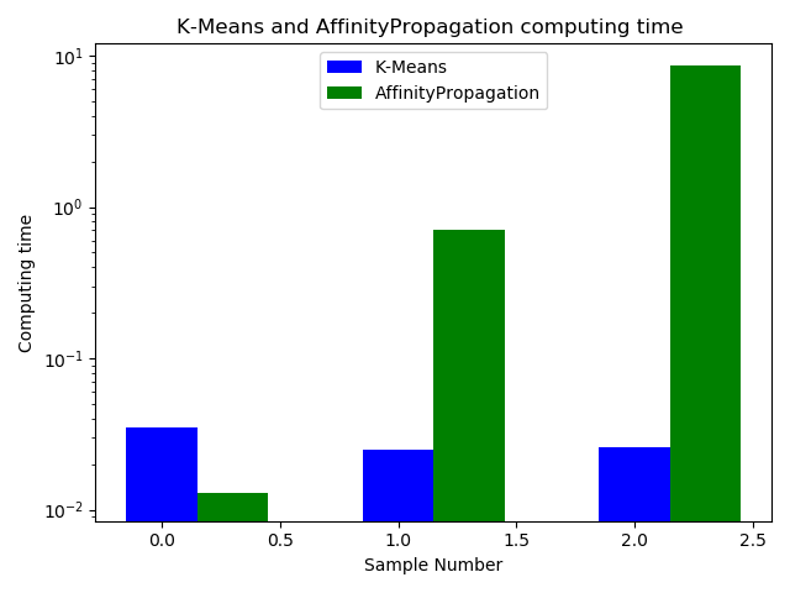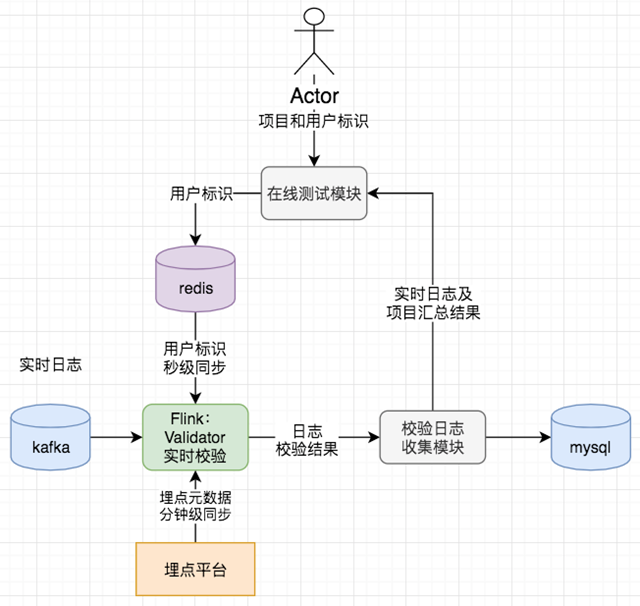##### 如何正确的进行数据埋点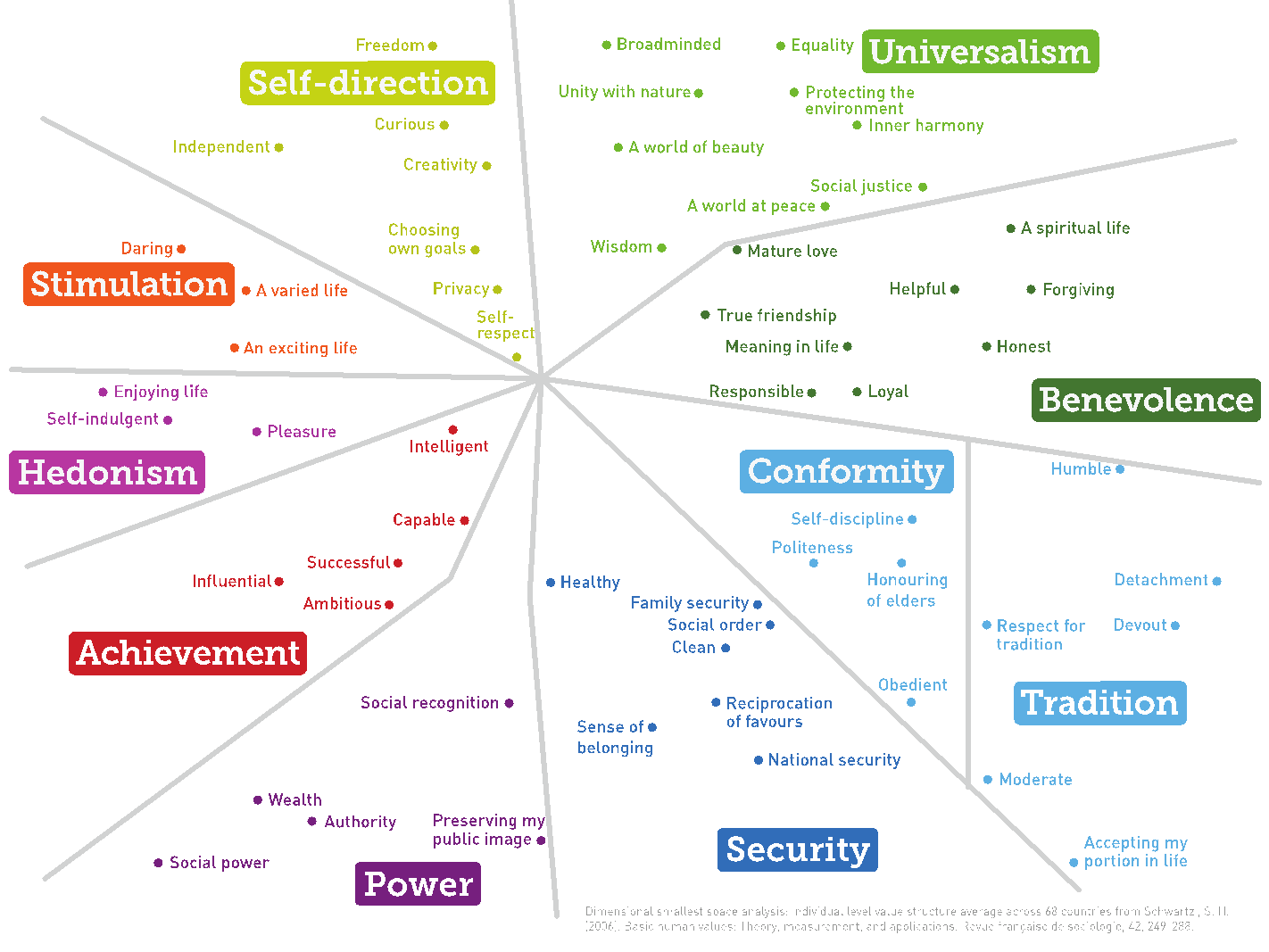##### 好的产品应有正确的价值观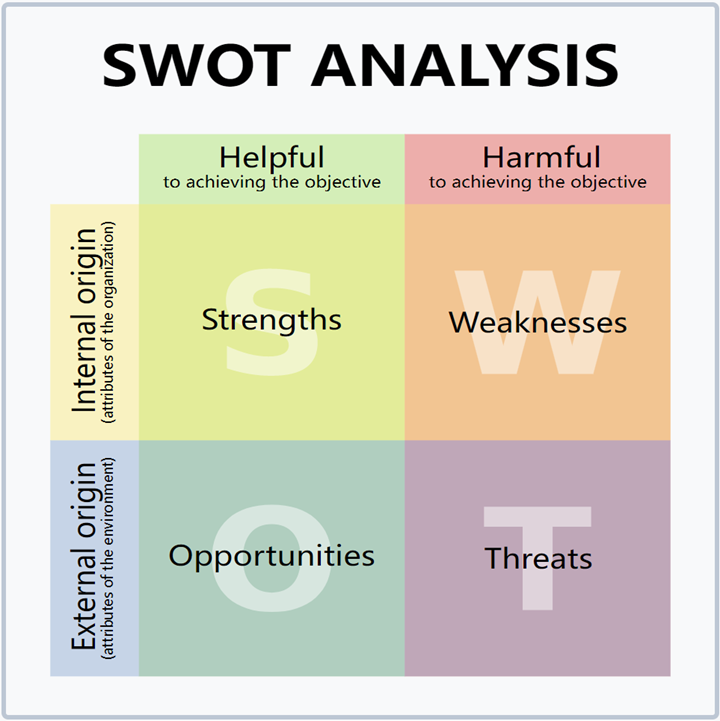## 2 Replies to “聚类算法之Affinity Propagation(AP)”

1. 蒋和平说道：

公式和原论文不太一样，请问是不是写错了呢